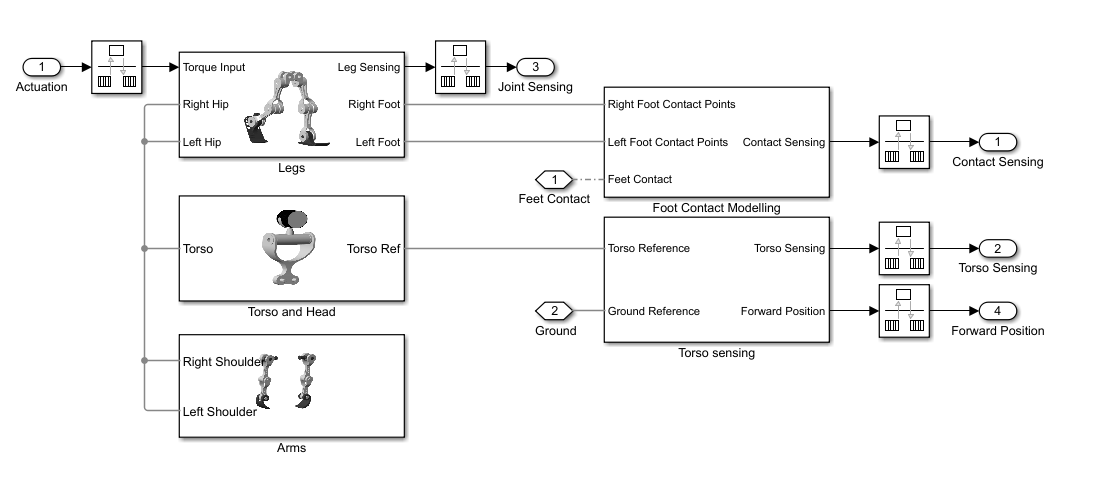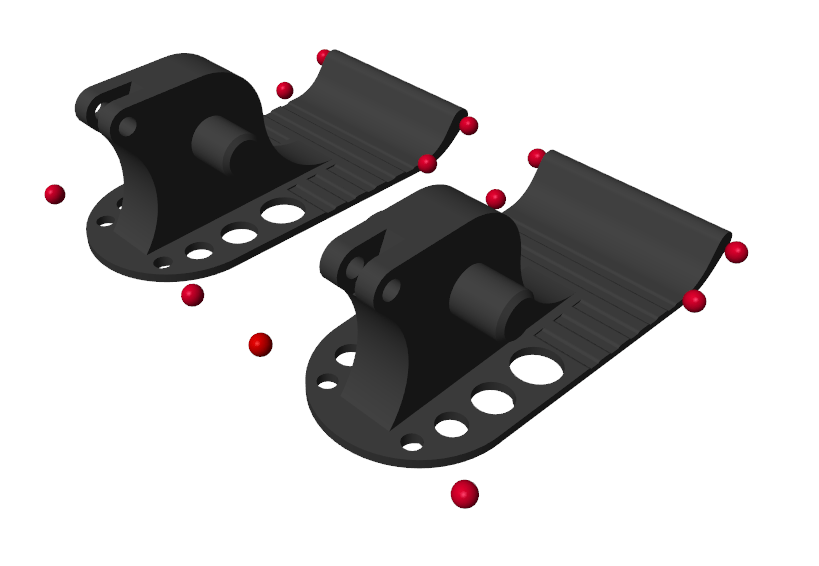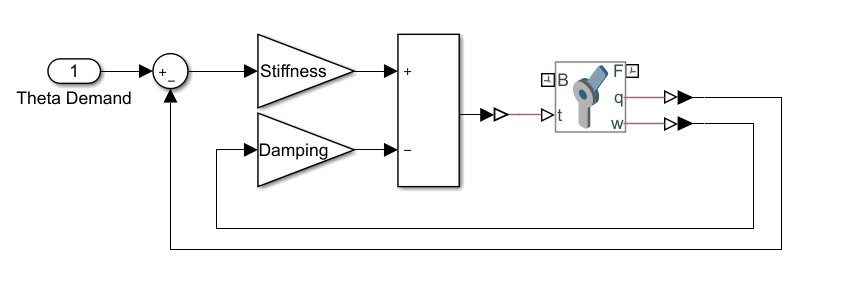# Train Humanoid Walker

This example shows how to model a humanoid robot using Simscape Multibody and train it using either a genetic algorithm (which requires a Global Optimization Toolbox license) or reinforcement learning (which requires Deep Learning Toolbox and Reinforcement Learning Toolbox licenses).

### Humanoid Walker Model

This example is based on a humanoid robot model. You can open the model by entering o`penExample("sm/ImportedURDFExample")` in the MATLAB® command prompt. Each leg of the robot has torque-actuated revolute joints in the frontal hip, knee, and ankle. Each arm has two passive revolute joints in the frontal and sagittal shoulder. During the simulation, the model senses the contact forces, position and orientation of the torso, joint states, and forward position. The figure shows the Simscape™ Multibody™ model on different levels.#### Contact Modeling

The model uses Spatial Contact Force (Simscape Multibody) blocks to simulate the contact between the feet and ground. To simplify the contact and speed up the simulation, red spheres are used to represent the bottoms of the robotic feet. For more details, see Use Contact Proxies to Simulate Contact (Simscape Multibody).#### Joint Controller

The model uses a stiffness-based feedback controller to control each joint . Model the joints as first-order systems with an associated stiffness (K) and damping (B), which you can set to make the joint behavior critically damped. The torque is applied when the setpoint ${\theta }_{0}$ differs from the current joint position $\theta$:

$\mathit{T}=\mathit{B}\stackrel{•}{\theta }+\mathit{K}\left({\theta }_{0}-\theta \right)$.

You can vary the spring set-point ${\theta }_{0}$ to elicit a feedback response to move the joint. The figure shows the Simulink model of the controller.### Humanoid Walker Training

The goal of this example is to train a humanoid robot to walk, and you can use various methods to train the robot. The example shows the genetic algorithm and reinforcement learning methods.

#### The Walking Objective Function

This example uses an objective function to evaluate different walking styles. The model gives a reward (${\mathit{r}}_{\mathit{t}}$) at each timestep:

`${\mathit{r}}_{\mathit{t}}={\mathit{w}}_{1\text{\hspace{0.17em}}}{\mathit{v}}_{\mathit{y}\text{\hspace{0.17em}}}+{\mathit{w}}_{2}{\mathit{t}}_{\mathit{s}}-{\mathit{w}}_{3\text{\hspace{0.17em}}}\mathit{p}-{\mathit{w}}_{4\text{\hspace{0.17em}}}\Delta \mathit{z}-{\mathit{w}}_{5\text{\hspace{0.17em}}}\Delta \mathit{x}$`

Here:

• ${\mathit{v}}_{\mathit{y}}$ — Forward velocity (rewarded)

• $\mathit{p}$ — Power consumption (penalized)

• $\Delta \mathit{z}$ — Vertical displacement (penalized)

• $\Delta \mathit{x}$ — Lateral displacement (penalized)

• ${\mathit{w}}_{1,...,5}$: Weights, which represent the relative importance of each term in the reward function

Additionally, not falling over is rewarded.

Consequently, the total reward ($\mathit{R}$) for a walking trial is:

`$\mathit{R}=\sum _{\mathit{t}=0}^{\mathit{T}}{\mathit{r}}_{\mathit{t}\text{\hspace{0.17em}}}$`

Here $\mathit{T}$ is the time at which the simulation terminates. You can change the reward weights in the `sm_humanoid_walker_rl_parameters` script. The simulation terminates when the simulation time is reached or the robot falls. Falling is defined as:

• The robot drops below 0.5 m.

• The robot moves laterally by more than 1 m.

• The robot torso rotates by more than 30 degrees.

#### Train with Genetic Algorithm

To optimize the walking of the robot, you can use a genetic algorithm. A genetic algorithm solves optimization problems based on a natural selection process that mimics biological evolution. Genetic algorithms are especially suited to problems when the objective function is discontinuous, nondifferentiable, stochastic, or highly nonlinear. For more information, see `ga` (Global Optimization Toolbox).The model sets the angular demand for each joint to a repeating pattern that is analogous to the central pattern generators seen in nature . The repeating pattern yields an open-loop controller. The periodicity of the signals is the gait period, which is the time taken to complete one full step. During each gait period, the signal switches between different angular demand values. Ideally, the humanoid robot walks symmetrically, and the control pattern for each joint in the right leg is transmitted to the corresponding joint in the left leg, with a delay of half a gait period. The pattern generator aims to determine the optimal control pattern for each joint and to maximize the walking objective function.To train the robot with a genetic algorithm, open the `sm_humanoid_walker_ga_train` script. By default, this example uses a pretrained humanoid walker. To train the humanoid walker, set `trainWalker` to `true`.

#### Train with Reinforcement Learning

Alternatively, you can also train the robot using a deep deterministic policy gradient (DDPG) reinforcement learning agent. A DDPG agent is an actor-critic reinforcement learning agent that computes an optimal policy that maximizes the long-term reward. DDPG agents can be used in systems with continuous actions and states. For details about DDPG agents, see `rlDDPGAgent` (Reinforcement Learning Toolbox).To train the robot with reinforcement learning, open the `sm_humanoid_walker_rl_train` script. By default, this example uses a pretrained humanoid walker. To train the humanoid walker, set `trainWalker` to `true`.

### References

 Kalveram, Karl T., Thomas Schinauer, Steffen Beirle, Stefanie Richter, and Petra Jansen-Osmann. “Threading Neural Feedforward into a Mechanical Spring: How Biology Exploits Physics in Limb Control.” Biological Cybernetics 92, no. 4 (April 2005): 229–40. https://doi.org/10.1007/s00422-005-0542-6.

 Jiang Shan, Cheng Junshi, and Chen Jiapin. “Design of Central Pattern Generator for Humanoid Robot Walking Based on Multi-Objective GA.” In Proceedings. 2000 IEEE/RSJ International Conference on Intelligent Robots and Systems (IROS 2000) (Cat. No.00CH37113), 3: 1930–35. Takamatsu, Japan: IEEE, 2000. https://doi.org/10.1109/IROS.2000.895253.